### Circles Class 9th Mathematics Part Ii MHB Solution

##### Circles Class 9th Mathematics Part Ii MHB Solution

###### Practice Set 6.1
Question 1.

Distance of chord AB from the center of a circle is 8 cm. Length of the chord AB is 12 cm. Find the diameter of the circle.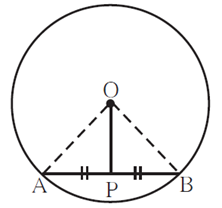Given that OP = 8 cm

And AB = 12 cm

We know that a perpendicular drawn from the center of a circle on its chord bisects

the chord.

∴ AP = PB = 6 cm

In the right angled ΔOAP using Pythagoras theorem,

⇒ OA2 = OP2 + AP2

⇒ OA2 = 82 + 62

⇒ OA2 = 64 + 36

⇒ OA2 = 100

⇒ OA = 10cm

So, the diameter of the circle is (2×10) = 20cm (Diameter = 2×Radius).

Question 2.

Diameter of a circle is 26 cm and length of a chord of the circle is 24 cm. Find the distance of the chord from the center.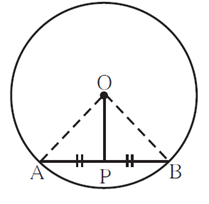Given that diameter = 26cm

Radius = Diameter / 2 = 26 /2 = 13cm

So, OA = 13cm

And AB = 24 cm

We know that a perpendicular drawn from the center of a circle on its chord bisects

the chord.

∴ AP = PB = 12 cm

In the right angled ΔOAP using Pythagoras theorem,

⇒ OA2 = OP2 + AP2

⇒ 132 = OP2 + 122

⇒ 169 = OP2 + 144

⇒ OP2 = 25

⇒ OP = 5cm

So, the distance of chord from the center is 5cm.

Question 3.

Radius of a circle is 34 cm and the distance of the chord from the center is 30 cm, find the length of the chord.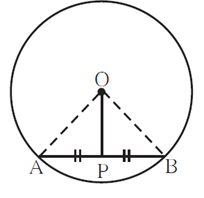Given that

So, OA = 34cm

And OP = 30 cm

We know that a perpendicular drawn from the center of a circle on its chord bisects

the chord.

∴ AP = PB,

AB = 2PB

In the right angled ΔOAP using Pythagoras theorem,

⇒ OA2 = OP2 + AP2

⇒ 342 = 302 + AP2

⇒ 1156 = 900 + AP2

⇒ AP2 = 256

⇒ AP = 16cm

So, the length of chord is 16× 2 = 32cm (AB = 2AP)

Question 4.

Radius of a circle with center O is 41 units. Length of a chord PQ is 80 units, find the distance of the chord from the center of the circle.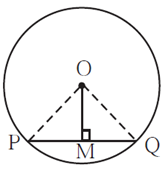Given that

So, OP = 41 units

And PQ = 80 units

We know that a perpendicular drawn from the center of a circle on its chord bisects

the chord.

∴ PM = MQ = 40 cm

In the right angled ΔOAP using Pythagoras theorem,

⇒ OP2 = OM2 + PM2

⇒ 412 = OM2 + 402

⇒ 1681 = OM2 + 1600

⇒ OM2 = 81

⇒ OM = 9 units

So, the distance of chord from the center is 9 units.

Question 5.

In figure 6.9, center of two circles is O. Chord AB of bigger circle intersects the smaller circle in points P and Q. Show that AP = BQ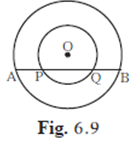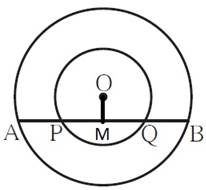We draw a perpendicular on chord AB from O.

We know that a perpendicular drawn from the center of a circle on its chord bisects

the chord.

Therefore,

AM = MB …….(1)

OM is also perpendicular to chord PQ of smaller circle.

Therefore,

PM = MQ ………….(2)

Subtracting (2) from (1)

AM-PM = MB-MQ

⇒ AP = BQ

Hence Proved.

Question 6.

Prove that, if a diameter of a circle bisects two chords of the circle then those two chords are parallel to each other.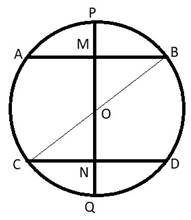We draw a circle with center O and AB, CD are the chords of this circle. Diameter PQ bisects AB and CD at M and N respectively.

We know that the line from the center bisecting the chord is perpendicular to the chord.

Therefore,

∠ OMA = ∠ OMB = 90°

Also, ∠ ONC = ∠ OND = 90°

∠ OMA + ∠ ONC = 90° + 90° = 180°

Hence the two chords, AB and CD are parallel to each other.

###### Practice Set 6.2
Question 1.

Radius of circle is 10 cm. There are two chords of length 16 cm each. What will be the distance of these chords from the center of the circle?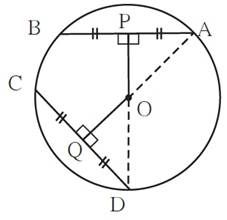Given radius of circle is 10cm

OA = OD = 10cm

AB = CD = 16cm

We know that a perpendicular drawn from the center of a circle on its chord bisects

the chord.

CQ = QD = 8cm

In right angled ΔOQD using the Pythagoras theorem

OD2 = OQ2 + QD2

102 = OQ2 + 82

100 = OQ2 + 64

OQ2 = 36

OQ = 6cm

Therefore the chord CD is at 6cm from the center.

We know that Congruent chords of a circle are equidistant from the center of the circle.

As AB and CD are equal in length, they are equidistant.

∴ OP = OQ = 6cm

Question 2.

In a circle with radius 13 cm, two equal chords are at a distance of 5 cm from the center. Find the lengths of the chords.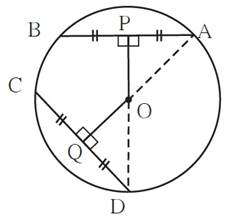Given radius of circle is 13cm

OA = OD = 13cm

OQ = OP = 16cm

We know that a perpendicular drawn from the centre of a circle on its chord bisects

the chord.

CQ = QD

CD = 2×QD

In right angled ΔOQD using the Pythagoras theorem

OD2 = OQ2 + QD2

132 = 52 + QD2

169 = 25 + QD2

QD2 = 144

QD = 12cm

Therefore the length of chord CD = 2×12 = 24cm

We know that The chords of a circle equidistant from the center of a circle are congruent

As AB and CD are equidistant, they are equal in length.

∴ AB = CD = 24cm

Question 3.

Seg PM and seg PN are congruent chords of a circle with center C. Show that the ray PC is the bisector of ∠NPM.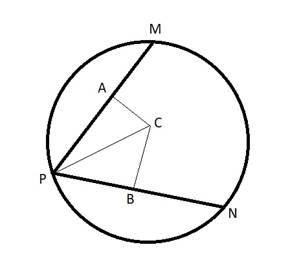Given that PM = PN

We know that Congruent chords of a circle are equidistant from the center of the circle.

Therefore, AC = CB ………………(1)

Also,

A perpendicular drawn from the centre of a circle on its chord bisects

the chord.

CB bisects PN as PB = BN,

Similarly, CA bisects PM as PA = AM.

In ΔAPC and ΔBPC,

∠CAP = ∠ CBP = 90°

PC = PC (common side)

AC = CB (From eq (1))

∴ ΔAPC≅ ΔBPC (RHS congruence)

∴ ∠ APC = ∠ BPC (by CPCT)

Hence proved that PC is the bisector of ∠ NPM.

###### Practice Set 6.3
Question 1.

Construct ΔABC such that ∠B = 1000, BC = 6.4, ∠C = 500 and construct its incircle.

Steps of Construction:

1.Construct ΔABC of given dimensions.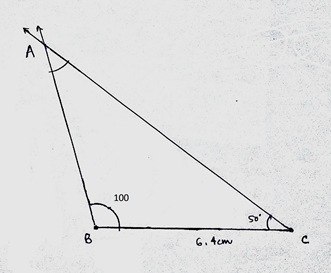2.Draw bisectors of two angles, ∠A and ∠B.

3.Denote the point of intersection as O.

4.Draw perpendicular OP on AB.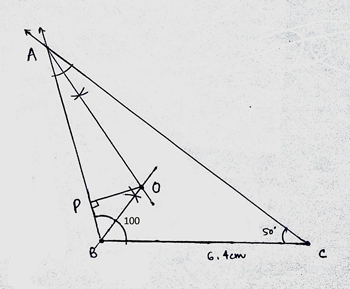5.Draw a circle with O as center and OP as radius.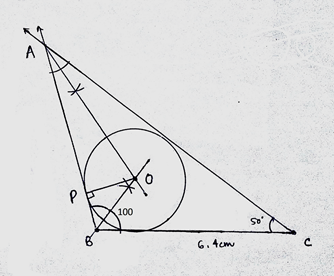Question 2.

Construct ΔPQR such that ∠700, ∠R = 500, QR = 7.3cm, and construct its circumcircle.

Steps of Construction:

1.Construct ΔPQR of given dimensions.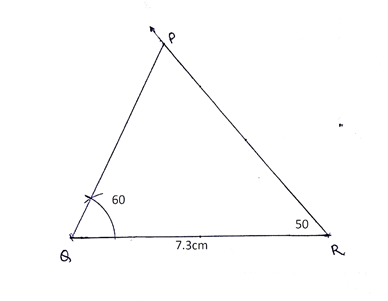2.Draw perpendicular bisectors of two sides, QR and PR.

3.Denote the point of intersection as O.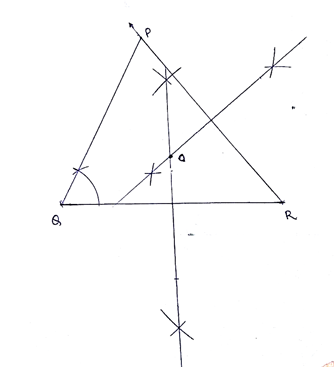4.Draw a circle with O as center and OP as radius.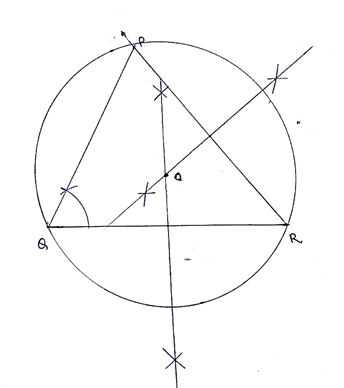Question 3.

Construct ΔXYZ such that XY = 6.7 cm, YZ = 5.8 cm, XZ = 6.9 cm. Construct its incircle.

Steps of Construction:

1.Construct ΔXYZ of given dimensions.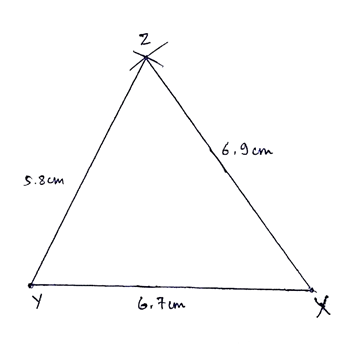2.Draw bisectors of two angles, ∠X and ∠Y.

3.Denote the point of intersection as O.

4.Draw perpendicular OA on XY.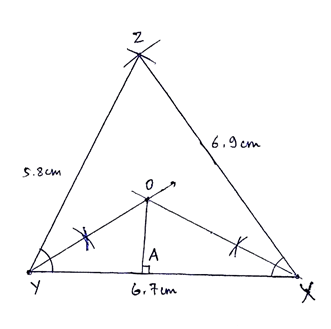5.Draw a circle with O as center and OA as radius.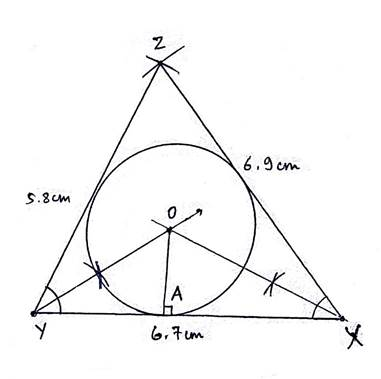Question 4.

In ΔLMN, LM = 7.2cm, ∠M = 1050, MN = 6.4cm, then draw ΔLMN and construct its circumcircle.

Steps of Construction:

1.Construct ΔLMN of given dimensions.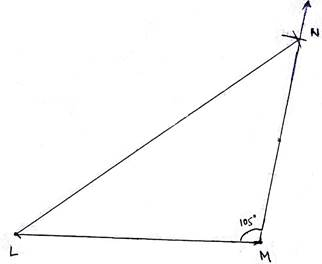2.Draw perpendicular bisectors of two sides, LM and MN.

3.Denote the point of intersection as O.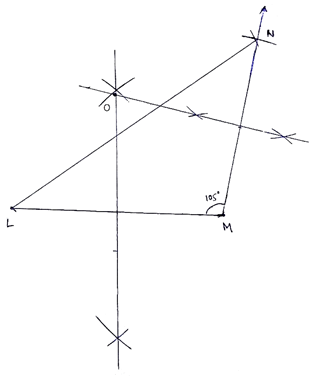4.Draw a circle with O as center and OM as radius.